# Lab 5B: Statistical Modeling (Hard)

1. Set the working directory

2. Load policies from the CSV file

### Problem 1: Create a Gaussian Distribution Model of Height

1. Create a plot of height (Centimeters)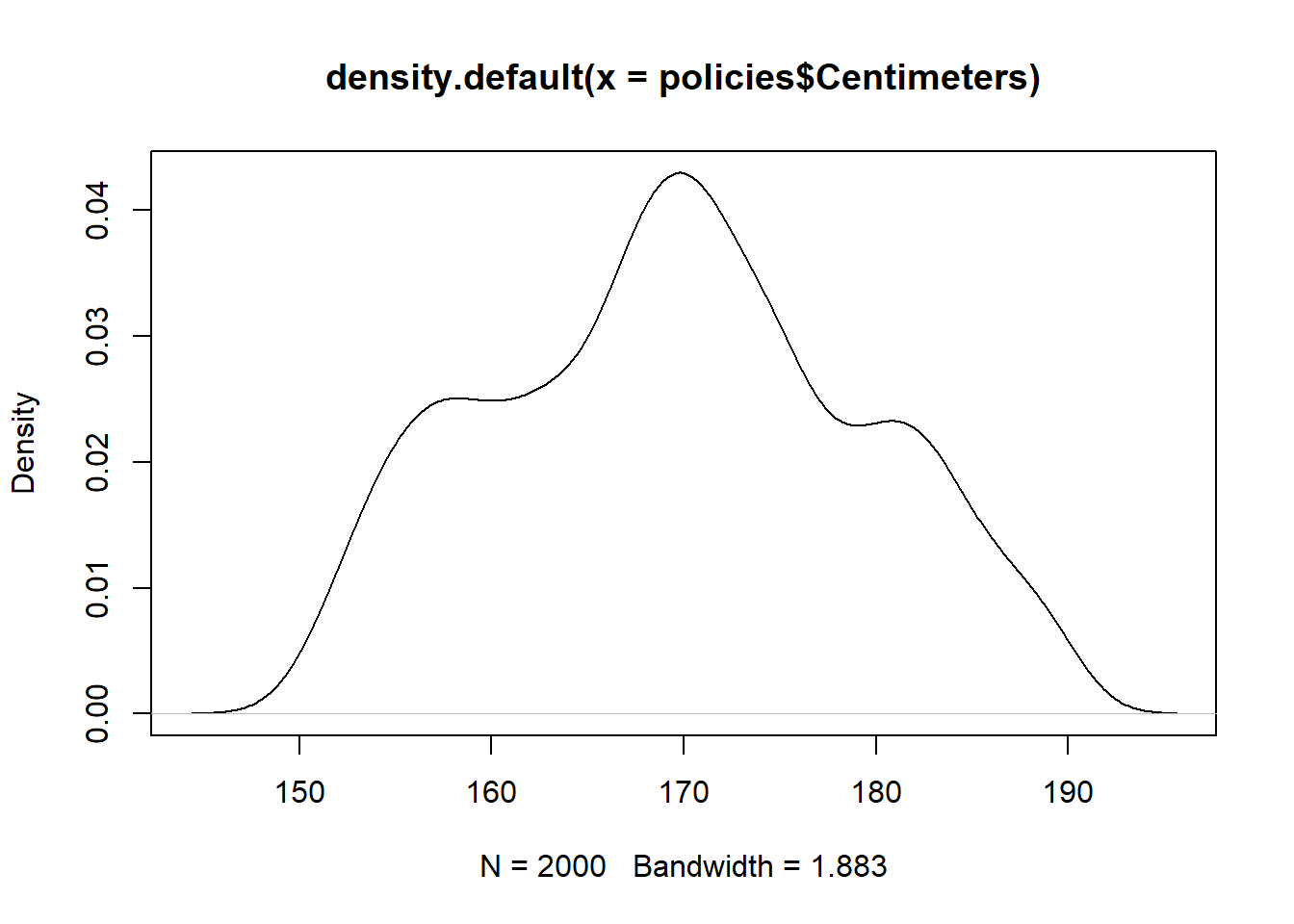2. Get the mean

3. Print the mean

##  169.632
1. Get the standard deviation

2. Print the standard devition

##  9.567358
1. Create points along x-axis of the distribution

2. Compute the y-axis height of each point

3. Add the distribution to the plot

plot(density(policies\$Centimeters))
lines(
x = distributionX,
y = distributionY,
col = "red")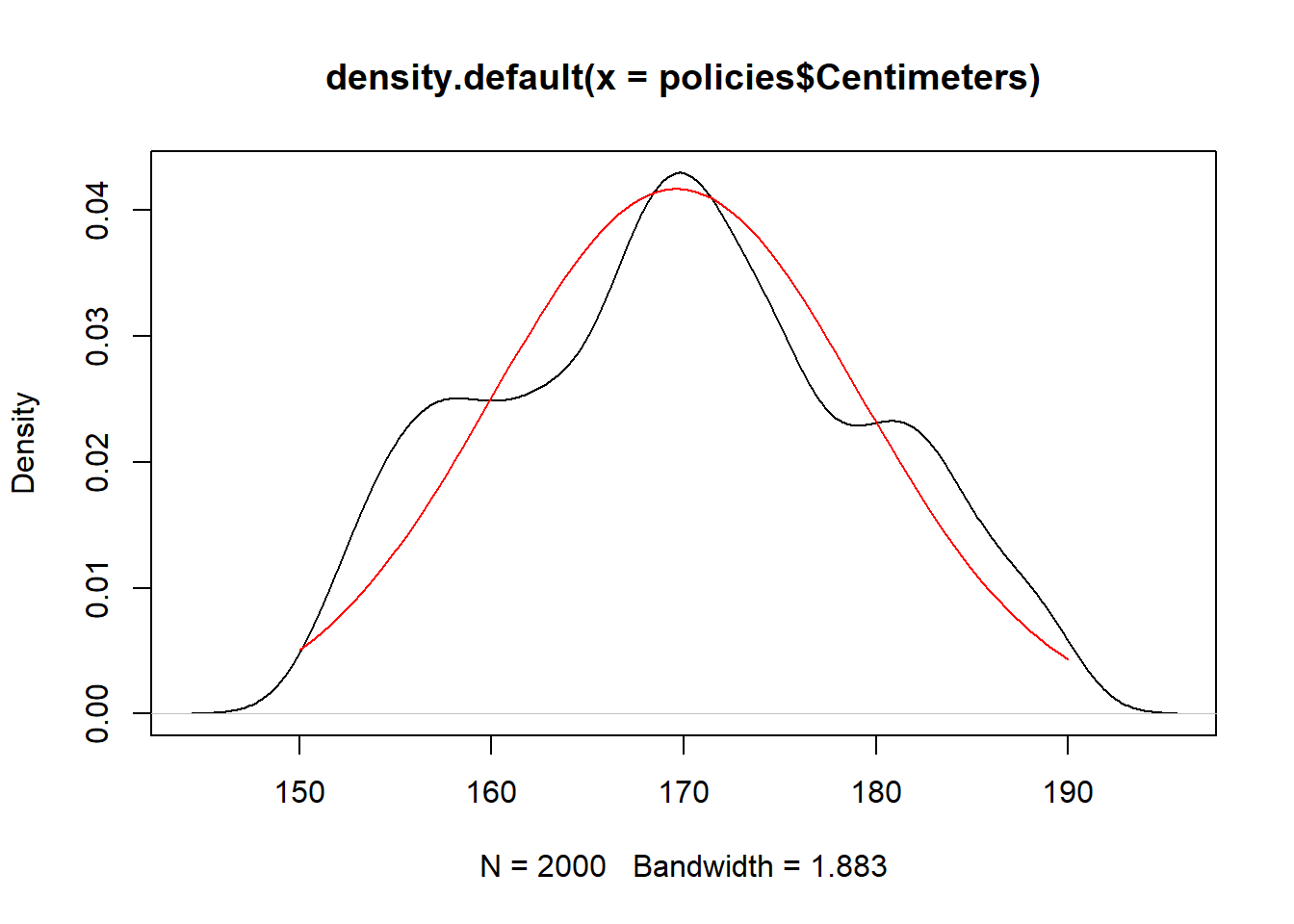1. Generate new values from the model

2. Add distribution of generated values to plot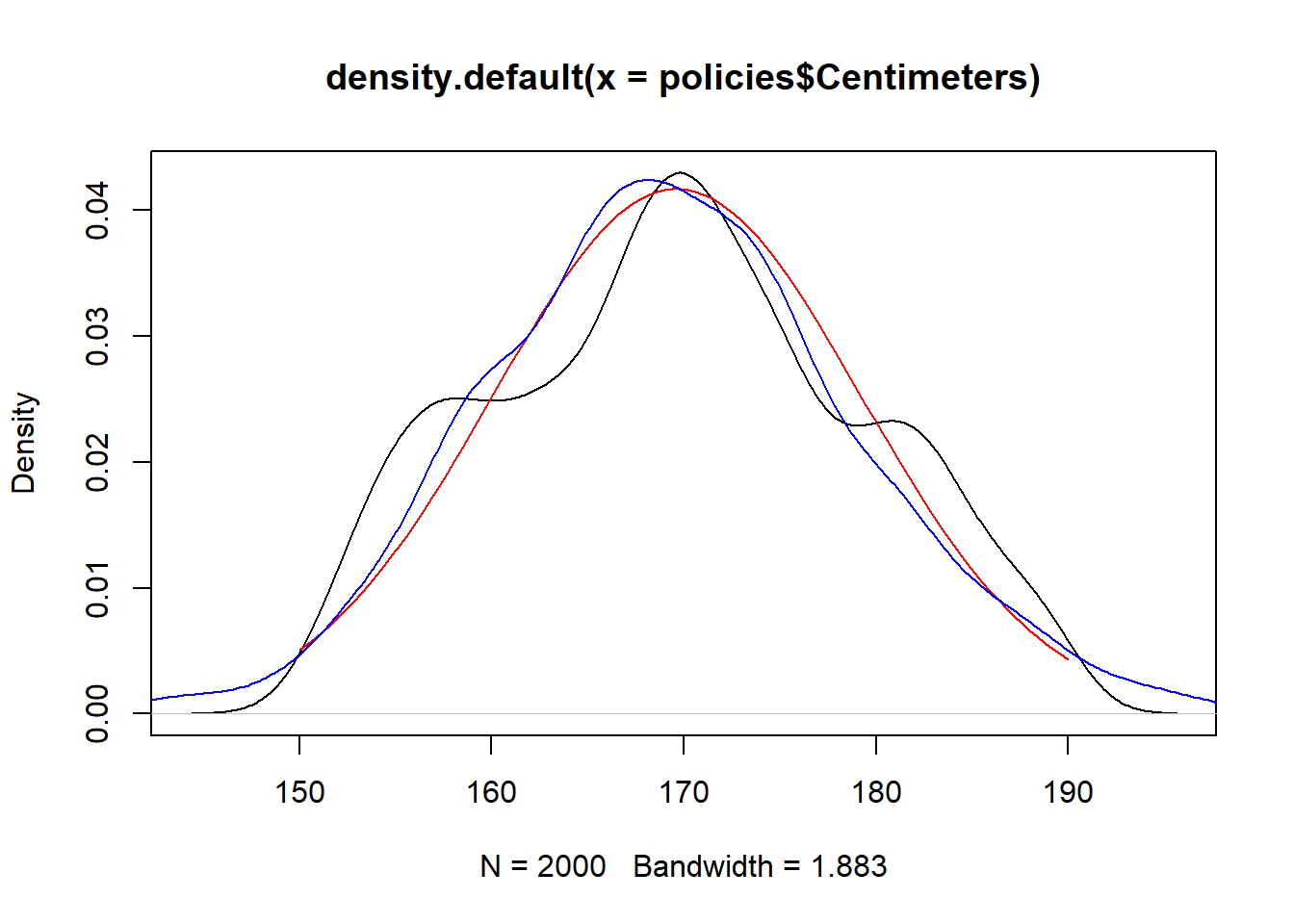3. Get the mean of the generated values

##  169.2888
1. Get the standard deviation of the generated values
##  9.590691

Question: What would happen to the mean and standard deviation if we increase n to 1,000,000?

### Problem 2: Create a Simple Linear Regression Model

1. Create a scatterplot of height (Centimeters) vs weight (Kilograms)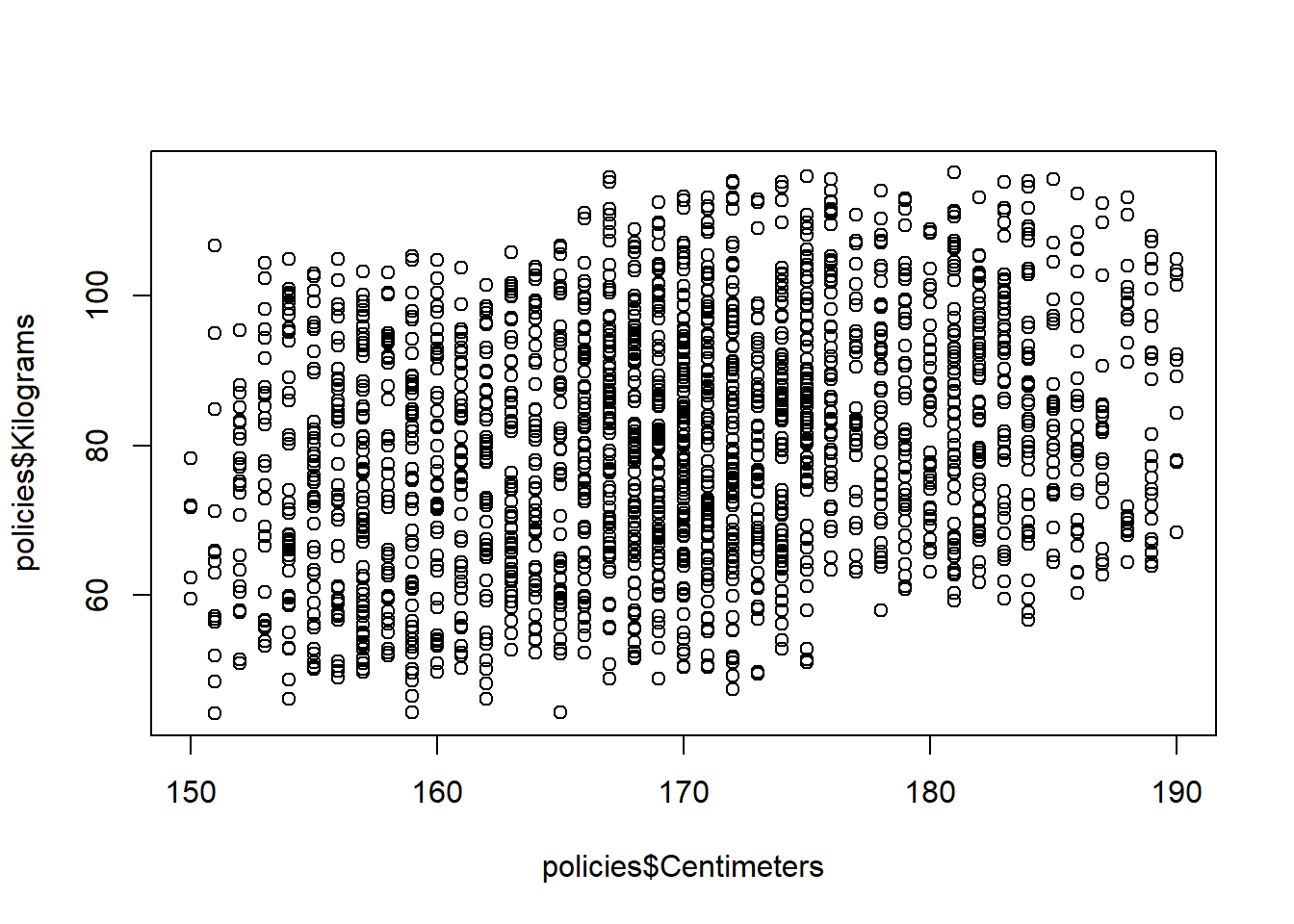2. Create a linear regression model

3. Draw the linear regression model on the plot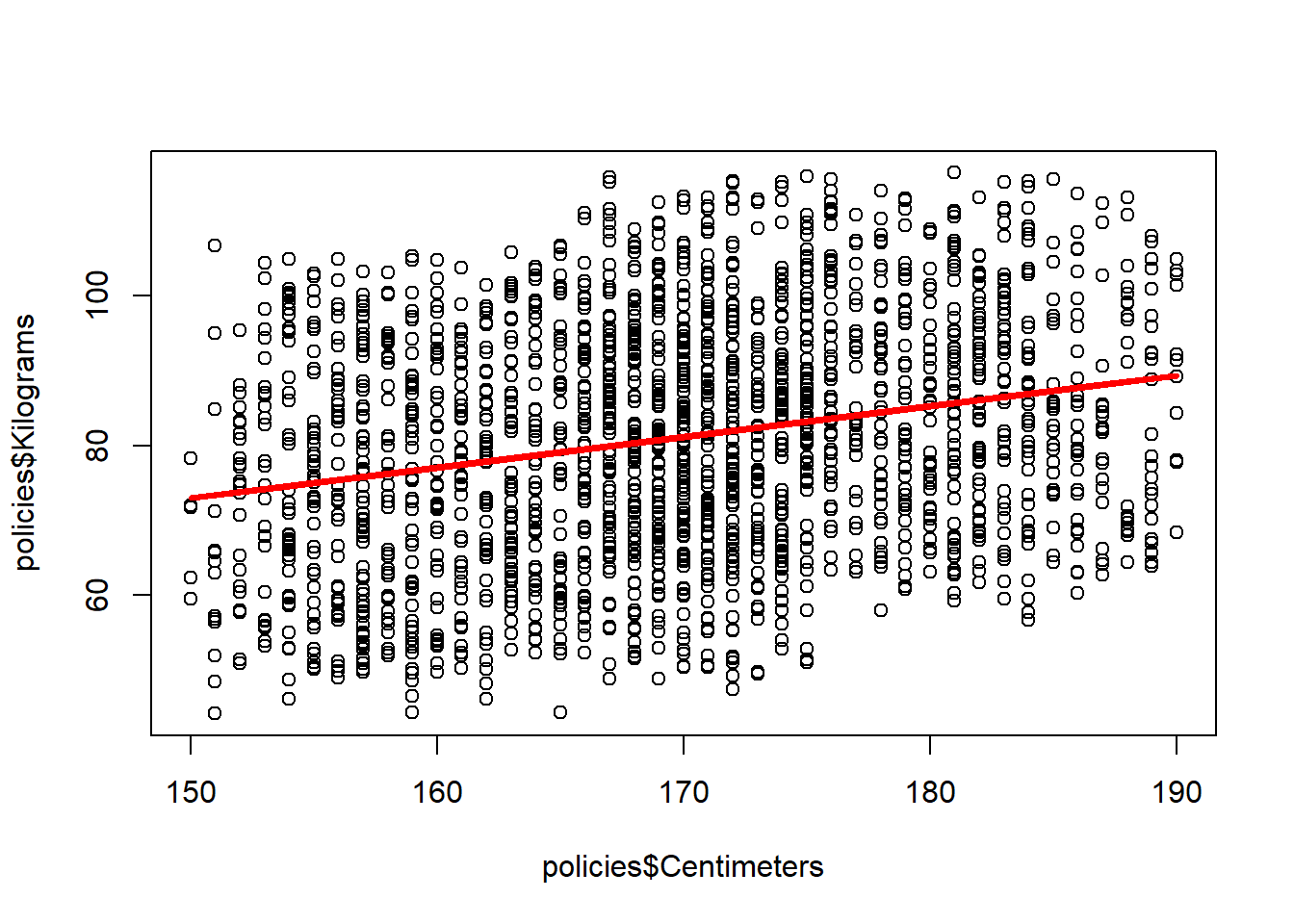4. Get the correlation coefficient

##  0.2467215
1. Summarize the model
##
## Call:
## lm(formula = Kilograms ~ Centimeters, data = policies)
##
## Residuals:
##     Min      1Q  Median      3Q     Max
## -34.914 -12.678  -0.038  12.247  35.962
##
## Coefficients:
##             Estimate Std. Error t value Pr(>|t|)
## (Intercept) 11.12801    6.15165   1.809   0.0706 .
## Centimeters  0.41204    0.03621  11.380   <2e-16 ***
## ---
## Signif. codes:  0 '***' 0.001 '**' 0.01 '*' 0.05 '.' 0.1 ' ' 1
##
## Residual standard error: 15.49 on 1998 degrees of freedom
## Multiple R-squared:  0.06087,    Adjusted R-squared:  0.0604
## F-statistic: 129.5 on 1 and 1998 DF,  p-value: < 2.2e-16
1. Create a table of unseen heights: 150, 175, 200

2. Predict new unknown weights based new unseen heights

##        1        2        3
## 72.93363 83.23457 93.53550

Question are their any problems with this linear regression model?

1. Create a scatterplot of Age vs Rate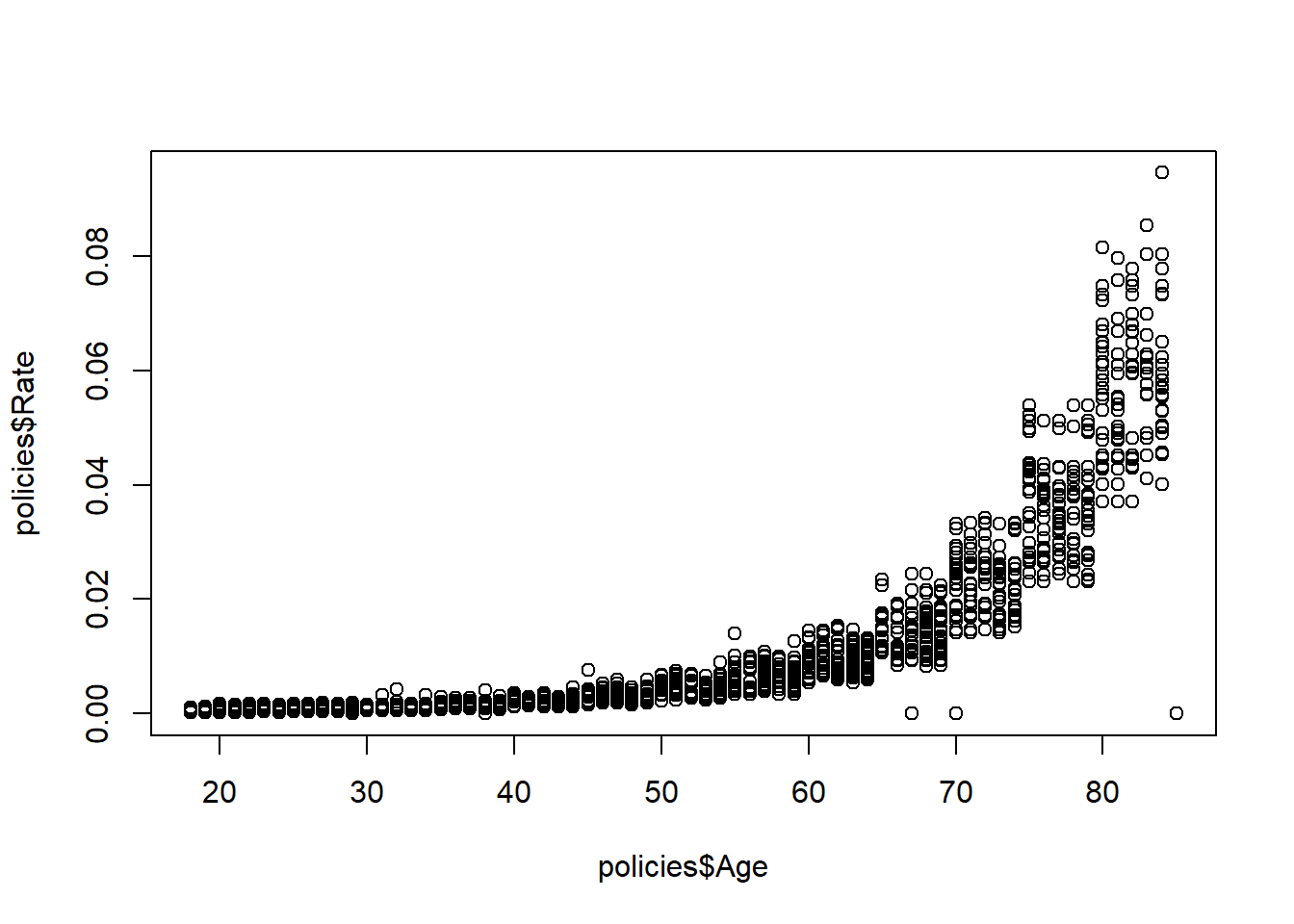2. Get the correlation coefficient

##  0.7387237

Question: Why is a linear model not a good model for these data?